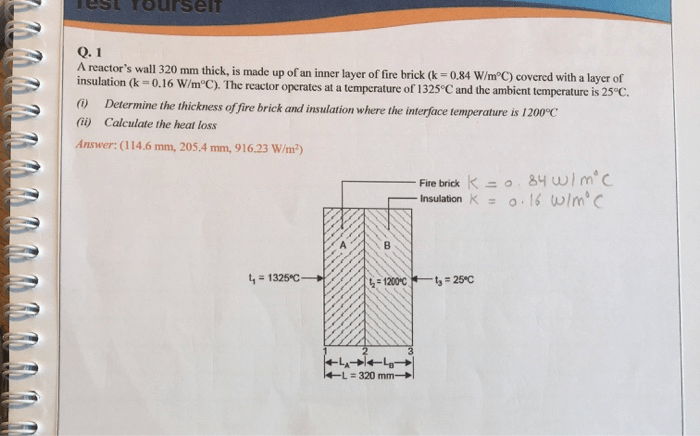# A reactor's wall 320 mm thick, is made up of an inner layer of fire brick (k = 0.84 W/m°C) covered with a layer of insulation (k -0.16 W/m°C). The reactor operates at a temperature of 1325°C and the ambient temperature is 25°C. () Determine the thickness of fire brick and insulation where the interface temperature is 1200°C (ii) Calculate the heat loss

Question-AnswerCategory: Heat And Mass TransferA reactor's wall 320 mm thick, is made up of an inner layer of fire brick (k = 0.84 W/m°C) covered with a layer of insulation (k -0.16 W/m°C). The reactor operates at a temperature of 1325°C and the ambient temperature is 25°C. () Determine the thickness of fire brick and insulation where the interface temperature is 1200°C (ii) Calculate the heat loss

A reactor’s wall 320 mm thick, is made up of an inner layer of fire brick (k = 0.84 W/m°C) covered with a layer of insulation (k -0.16 W/m°C). The reactor operates at a temperature of 1325°C and the ambient temperature is 25°C. () Determine the thickness of fire brick and insulation where the interface temperature is 1200°C (ii) Calculate the heat loss